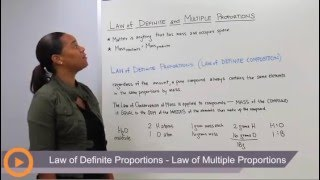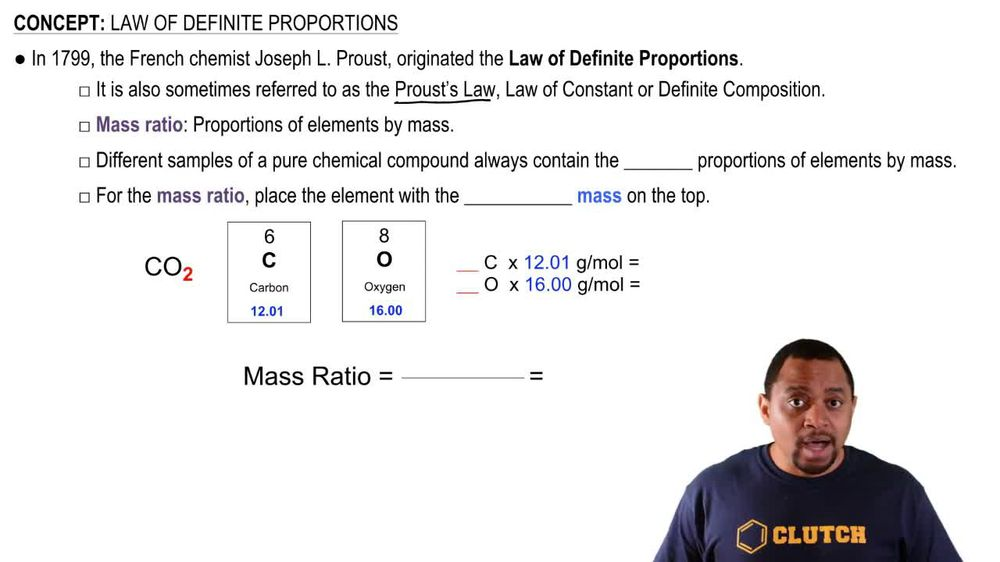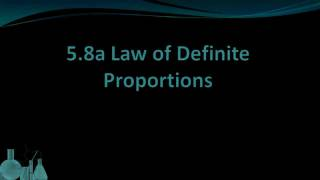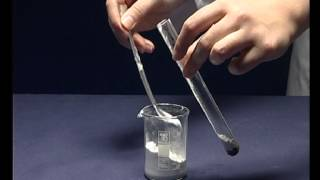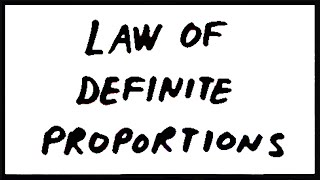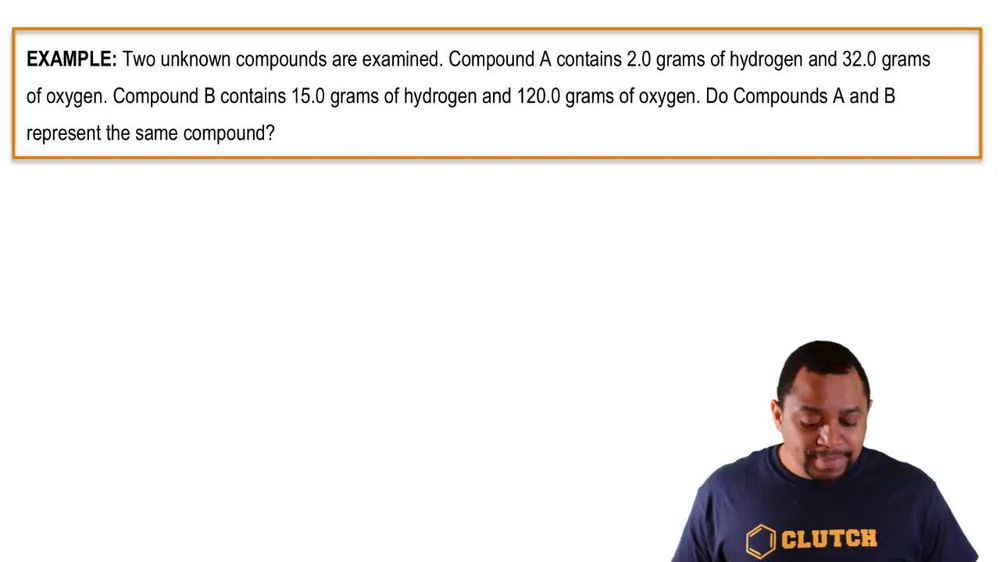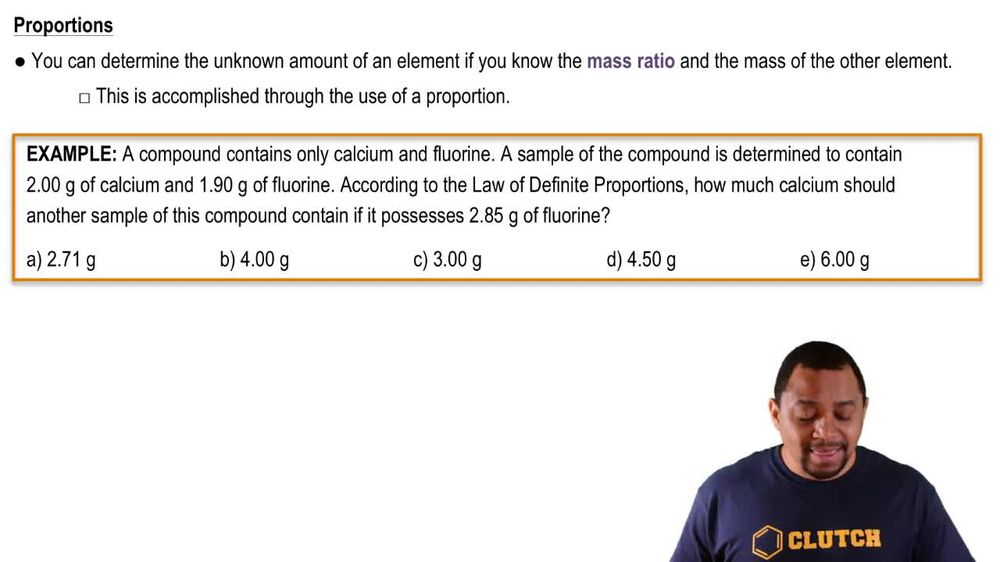Start typing, then use the up and down arrows to select an option from the list.
1. 2. Atoms & Elements2. Law of Definite Proportions
Problem

# Two samples of carbon tetrachloride are decomposed into their constituent elements. One sample produces 38.9 g of carbon and 448 g of chlorine, and the other sample produces 14.8 g of carbon and 134 g of chlorine. Are these results consistent with the law of definite proportions? Explain your answer.

Relevant Solution3m
Play a video:
everyone in this example, we have two samples that are going through decomposition forming sodium and chlorine. And we need to figure out which values below will be consistent with the law of definite proportions. So our first step is to recall with the law of definite proportions, tells us, and we should recall that it tells us that the ratio of our elements must remain consistent. So there will be no ratio change. And so what that means is that looking at the given ratio for the first sample, we have four 26 g of sodium. Sorry, that should be a point there. So they tell us we have 4.26 g of sodium To 6.57 g of chlorine. So we want a similar ratio Also for the second sample. And so we want to find the value of this ratio. So when we divide 4.26g divided by 6.57 For a sample one We get a value in our calculators equal to 0.6484. So we want the same value for our ratio for the second sample. And so what we're going to do is look at the answer choices and define the ratios from them. So for answer choice A. We have 8.52 g of sodium for 13.14 g of chlorine. And so this ratio value gives us 0.6484. Which is actually very good because this is what we want. So we can definitely consider this a potential correct choice. Moving onto choice B. We have 8.52 g of sodium for 6. g of chlorine. And so this gives us a value of 1.296. So we can rule out choice B. Because 1.296 is not matching up with the ratio that we want 0.6484 as the value. So moving on to choice C. We have 4.26 g of chlorine For 13. g of chlorine, sorry, of sodium to chlorine. And so this value here gives us 0.30-42. So we can also rule out choice. See lastly, we have choice D. Where we have 4.26 g of sodium and 6.57 g of chlorine. So this sample did not even undergo another decomposition. And so we would rule out choice D. Because the ratio will have not increased in any way or not. The ratio but the masses of our compounds did not increase in anyway. So we're going to just go ahead and confirm that choice A. Is the correct choice. That is consistent with the law of definite proportions. And that is because we maintained the same proportion of our ratio value that we should have had from our original sample for the given sample in choice. A. So I hope that everything we reviewed was clear. But if you have any questions, please leave them down below. And I will see everyone in the next practice video.